# How To Find The Next Row in VBA Microsoft Excel 2010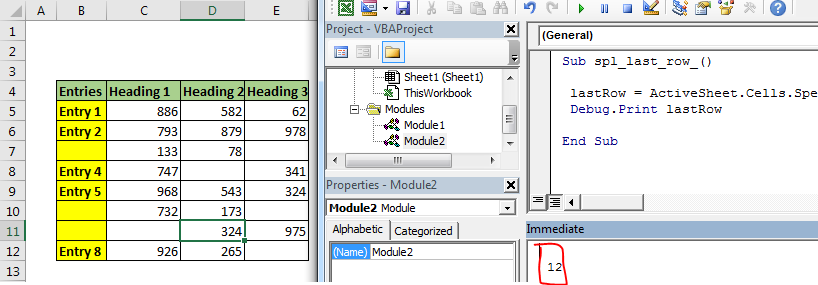Finding the next row means finding the last used row +1. Finding the last used row or column is one of the basic and important tasks for any automation in excel using VBA. For compiling sheets, workbooks and arranging data automatically, you are required to find the limit of the data on sheets.

This article will explain every method of finding the last row and column in excel in the easiest ways.

#### 1. Find Last Non-Blank Row in a Column using Range.End

Let’s see the code first. I’ll explain the letter.

```Sub getLastUsedRow()

Dim last_row As Integer

last_row = Cells(Rows.Count, 1).End(xlUp).Row ‘This line gets the last row

Debug.Print last_row

End Sub

```

The above sub finds the last row in column 1.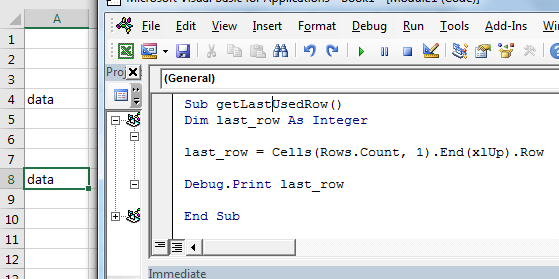How does it work?

It is just like going to the last row in a sheet and then pressing CTRL+UP shortcut.

Cells(Rows.Count, 1): This part selects the cell in column A.  Rows.Count gives 1048576, which is usually the last row in excel sheet.

Cells(1048576, 1).End(xlUp): End is a method of range class which is used to navigate in sheets to ends. xlUp is the variable that tells the direction. Together this command selects the last row with data.

Cells(Rows.Count, 1).End(xlUp).Row : .row returns the row number of selected cell. Hence we get the row number of the last cell with data in column A. in our example, it is 8.

See how easy it is to find last rows with data. This method will select the last row in data irrespective of blank cells before it. You can see that in image that only cell A8 has data. All preceding cells are blank except A4.

#### Select the last cell with data in a column

If you want to select the last cell in A column then just remove “.row” from the end and write .select.

```Sub getLastUsedRow()

Cells(Rows.Count, 1).End(xlUp).Select ‘This line selects the last cell in a column

End Sub

```

The “.Select” command selects the active cell.

#### Get last cell’s address column

If you want to get the last cell’s address in A column then just remove “.row” from the end and write .address.

```Sub getLastUsedRow()

End Sub

```

#### Find Last Non-Blank Column in a Row

It is almost the same as finding the last non blank cell in a column. Here we are getting the column number of the last cell with data in row 4.

```Sub getLastUsedCol()

Dim last_col As Integer

last_col = Cells(4,Columns.Count).End(xlToLeft).Column ‘This line gets the last column

Debug.Print last_col

End Sub
```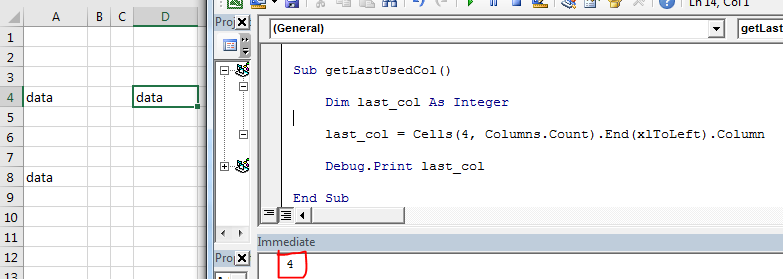You can see in the image that it is returning the last non blank cell’s column number in row 4. Which is 4.

How it works?

Well, the mechanics are the same as finding the last cell with data in a column. We just have used keywords related to columns.

#### Select Data Set in Excel Using VBA

Now we know how to get the last row and last column of excel using VBA. Using that we can select a table or dataset easily. After selecting a data set or table, we can do several operations on them, like copy-paste, formatting, deleting etc.

Here we have a data set. This data can expand downwards. Only the starting cell is fixed, which is B4. The last row and column is not fixed.  We need to select the whole table dynamically using vba.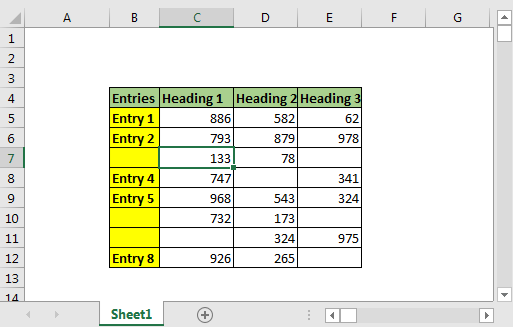VBA code to select table with blank cells

```Sub select_table()
Dim last_row, last_col As Long

'Get last row
last_row = Cells(Rows.Count, 2).End(xlUp).Row

'Get last column
last_col = Cells(4, Columns.Count).End(xlToLeft).Column

'Select entire table
Range(Cells(4, 2), Cells(last_row, last_col)).Select

End Sub
```

When you run this, the entire table will be selected in a fraction of a second. You can add new rows and columns. It will always select the entire data.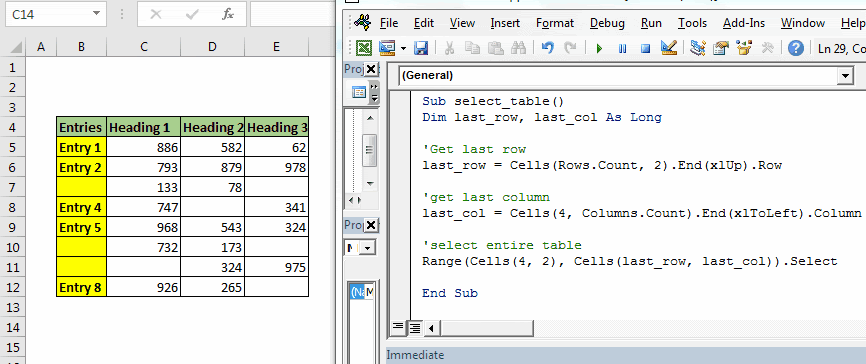Benefits of this method:

1. It’s easy. We literally wrote only one line to get the last row with data. This makes it easy.
2. Fast. Less line of code, less time taken.
3. Easy to understand.
4. Works perfectly if you have a clumsy data table with fixed starting points.

Cons of Range.End method:

1. The starting point must be known.
2. You can only get the last non-blank cell in a known row or column. When your starting point is not fixed, it will be useless. Which is very less likely to happen.

#### 2. Find Last Row Using Find() Function

Let’s see the code first.

```Sub last_row()

lastRow = ActiveSheet.Cells.Find("*", searchorder:=xlByRows, searchdirection:=xlPrevious).Row

Debug.Print lastRow

End Sub
```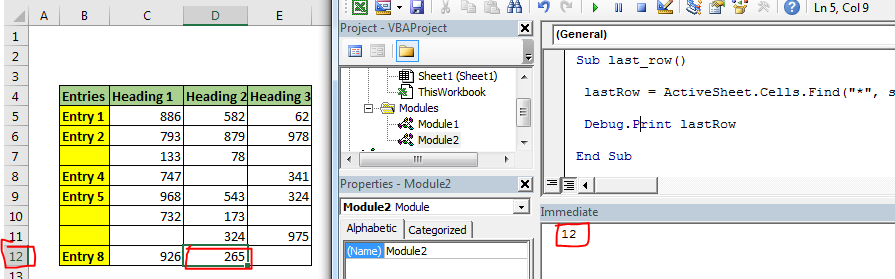As you can see in the image, this code returns the last row accurately.

#### How does it work?

Here we use find function to find any cell that contains any thing using wild card operator "*". Asterisk is used to find anything, text or number.

We set search order by rows (searchorder:=xlByRows). We also tell excel vba the direction of search as xlPrevious (searchdirection:=xlPrevious). It makes a find function to search from the end of the sheet, row wise.

Once it finds a cell that contains anything, it stops. We use the Range.Row method to fetch the last row from the active cell.

Benefits of Find function for getting last cell with data:

1. You don’t need to know the starting point. It just gets you last row.
2. It can be generic and can be used to find the last cell with data in any sheet without any changes.
3. Can be used to find any last instance of specific text or number on sheet.

Cons of Find() function:

1. It is ugly. Too many arguments.
2. It is slow.
3. Can’t get to the last non blank column. Technically, you can. But it gets too slow.

### 3. Using SpecialCells Function To Get Last Row

The SpecialCells function with xlCellTypeLastCell argument returns the last used cell. Lets see the code first

```Sub spl_last_row_()

lastRow = ActiveSheet.Cells.SpecialCells(xlCellTypeLastCell).Row
Debug.Print lastRow

End Sub
```If you run the above code, you will get the row number of the last used cell.

How it Works?

This is the vba equivalent of shortcut CTRL+End in excel. It selects the last used cell.  If you record the macro while pressing CTRL+End, you will get this code.

```Sub Macro1()
'
' Macro1 Macro
'

'
ActiveCell.SpecialCells(xlLastCell).Select
End Sub
```

We just used it to get the last used cell’s row number.

Note: As I said above, this method will return the last used cell not last cell with data. If you delete the data in the last cell, above vba code will still return the same cell reference, since it was the “last used cell”. You need to save the document first to get the last cell with data using this method.

Hope this article about How To Find The Next Row in VBA Microsoft Excel 2010 is explanatory. Find more articles on IF condition formulas here. If you liked our blogs, share it with your fristarts on Facebook. And also you can follow us on Twitter and Facebook. We would love to hear from you, do let us know how we can improve, complement or innovate our work and make it better for you. Write to us at info@exceltip.com.

Related Articles:

Getting Started With Excel VBA UserForms : I will explain how to create a form in excel, how to use VBA toolbox, how to handle user inputs and finally how to store the user inputs. We will go through these topics using one example and step by step guide.

VBA variables in Excel : VBA stands for Visual Basic for Applications. It is a programming language from Microsoft. It is used with Microsoft Office applications such as MSExcel, MS-Word and MS-Access whereas VBA variables are specific keywords.

Excel VBA Variable Scope : In all the programming languages, we have variable access specifiers that define from where a defined variable can be accessed. Excel VBA is no Exception. VBA too has scope specifiers.

ByRef and ByVal Arguments : When an argument is passed as a ByRef argument to a different sub or function, the reference of the actual variable is sent. Any changes made into the copy of the variable, will reflect in the original argument.

Popular Articles :

How to use the IF Function in Excel : The IF statement in Excel checks the condition and returns a specific value if the condition is TRUE or returns another specific value if FALSE.

How to use the VLOOKUP Function in Excel : This is one of the most used and popular functions of excel that is used to lookup value from different ranges and sheets.

How to use the SUMIF Function in Excel : This is another dashboard essential function. This helps you sum up values on specific conditions.

How to use the COUNTIF Function in Excel : Count values with conditions using this amazing function. You don't need to filter your data to count specific values. Countif function is essential to prepare your dashboard.

1.